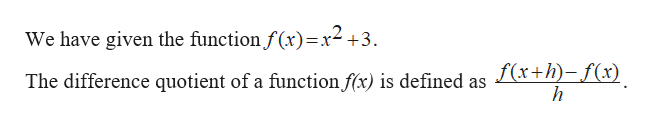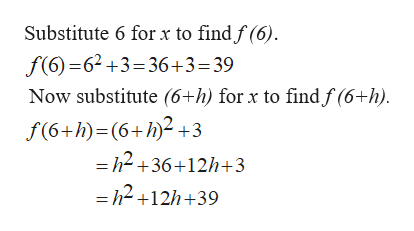# Evaluate the difference quotient for the given function. Simplify your answer.f(6 h) f(6)hf(x) x2 3,= XXSubmit Answer

Question
9 views

f(x) = x2 + 3,

 f(6 + h) − f(6) h

Evaluate the differene quiotent for the given fucntion and simplfy your answer.

check_circle

Step 1help_outlineImage TranscriptioncloseWe have given the function f (x)=x2+3 f(x+h)-f(x) h The difference quotient of a function f(x) is defined as fullscreen
Step 2help_outlineImage TranscriptioncloseSubstitute 6 for x to find f (6). f(6) 62+3 36+3=39 Now substitute (6+h) for x to findf (6+h) f(6+h) (6+h)2+3 =h2 +36+12h+3 =h2 +12h+ 39 fullscreen
Step 3

Thus the difference quotient fo...

### Want to see the full answer?

See Solution

#### Want to see this answer and more?

Solutions are written by subject experts who are available 24/7. Questions are typically answered within 1 hour.*

See Solution
*Response times may vary by subject and question.
Tagged in

### Other